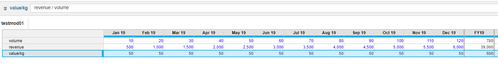# Summary Settings in module Blueprint viewedited November 2022 in Modeling

I'd like to know the difference of Summary settings combination in the blueprint view between:
Summary- Formula, Time Summary: Same as main summary
vs
Summary-None, Time Summary: Formula

If possible can anyone explain me with a small sample data like this:Tagged:

•edited November 2022

In Anaplan,

For a Number formatted line item the default Summary setting is "Sum".

For Text, List & Boolean formatted line item it is "None"

That means if there is any flat list (with top level set) or composite hierarchy as a dimension in the module then the numbers are rolled up automatically from granular level to the top level. On the other hand if there is a module dimensioned by Time as well it has its own settings.

Summary- Formula, Time Summary: Same as main summary - This means that the data will be calculated on aggregated levels as well as opposed to default Summation for Hierarchical Lists. If Time is set as "Same as Main Summary" then it means this is also kept as Formula. Your Summary Settings can also be read as Summary - Formula, Time - Formula.

Summary- None, Time Summary: Formula-  This means that the data will NOT be aggregated at higher levels of the hierarchy. On the other hand, it will calculate the data as per the formula for TIME dimension.

Note:If you want to see it working, I would recommend you add a hierarchical list as a dimension to your module and also keep Time in it.

Hope that helps,

Misbah

Miz Logix

•edited November 2022

@Misbah
Thank you for the lucid explanation, 👍